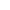Go to content
To work out the sum of two large numbers:
• set the numbers out in columns
• line up the units digits
• start by adding the units digits
• then work across to the leftYou can get a better display of the maths by downloading special TeX fonts from jsMath. In the meantime, we will do the best we can with the fonts you have, but it may not be pretty and some equations may not be rendered correctly.

Glossary

union

The union of two sets A and B is the set containing all the elements of A and B.

work

Equal to F x s, where F is the force in Newtons and s is the distance travelled and is measured in Joules.

Full Glossary List

This question appears in the following syllabi:

SyllabusModuleSectionTopicExam Year
AQA GCSE (9-1) Foundation (UK)N: Structure and CalculationN2: Four Operations and Place ValueAdding and Subtracting-
CIE IGCSE (9-1) Maths (0626 UK)1 NumberC1.8 Four Rules and PrecedenceAdding and Subtracting-
Edexcel GCSE (9-1) Foundation (UK)N: Structure and CalculationN2: Four Operations and Place ValueAdding and Subtracting-